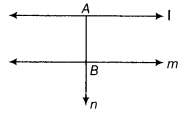# In the given figure, line l is parallel to line m. Prove that line n

In the given figure, line l is parallel to line m. Prove that line n, which is perpendicular to line l at A, is also perpendicular to the line m at B.Now, ∠1 = ∠2 = 90°.
Let if possible n is not perpendicular to m at B.
Then, either ∠3 or ∠4 is less than 90°.
Let us take ∠3 is less than 90°.
This implies∠1 + ∠3 < 180°.
Then, by Euclid’s fifth postulate, lines l and m intersect.
But we are given that l || m.
Thus, our assumption is wrong.
∴ n is perpendicular to m at B.